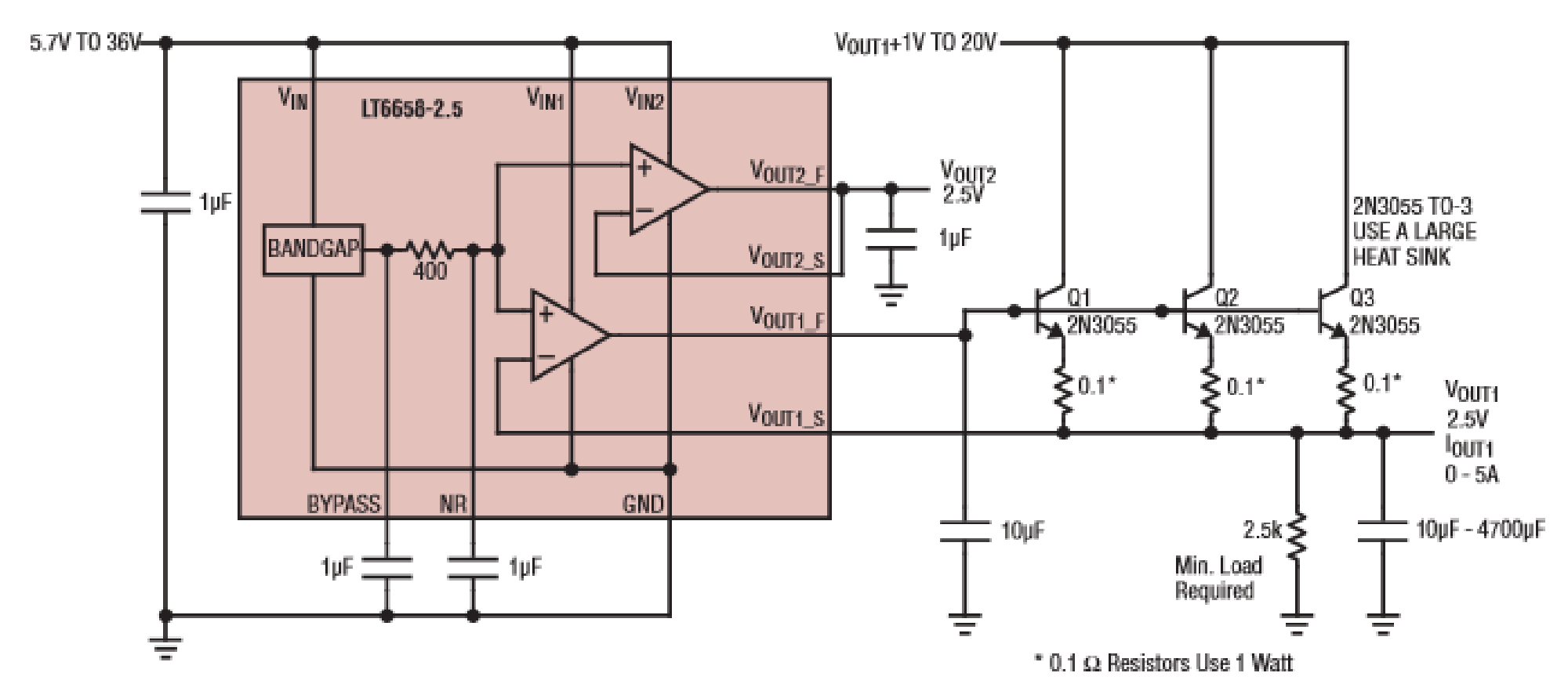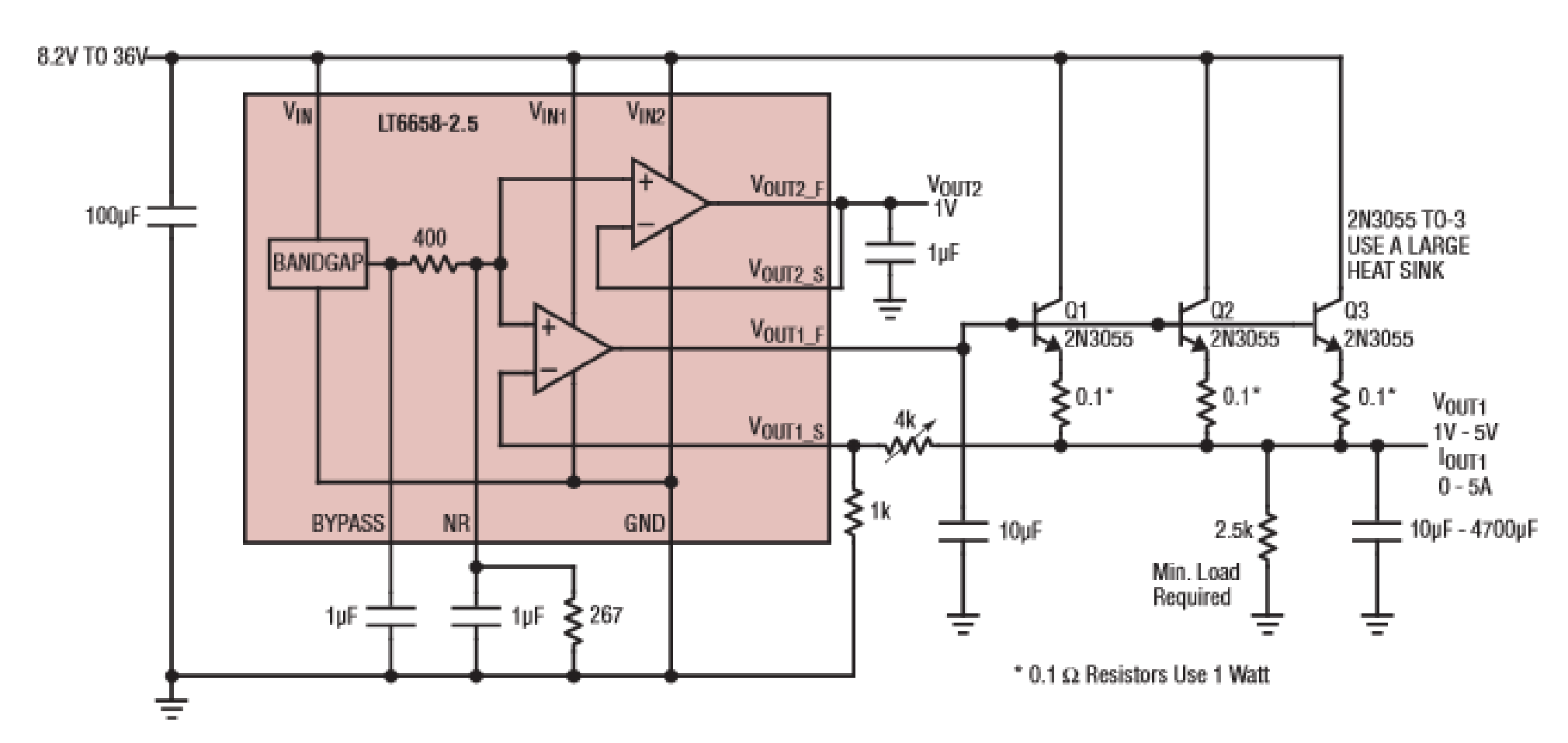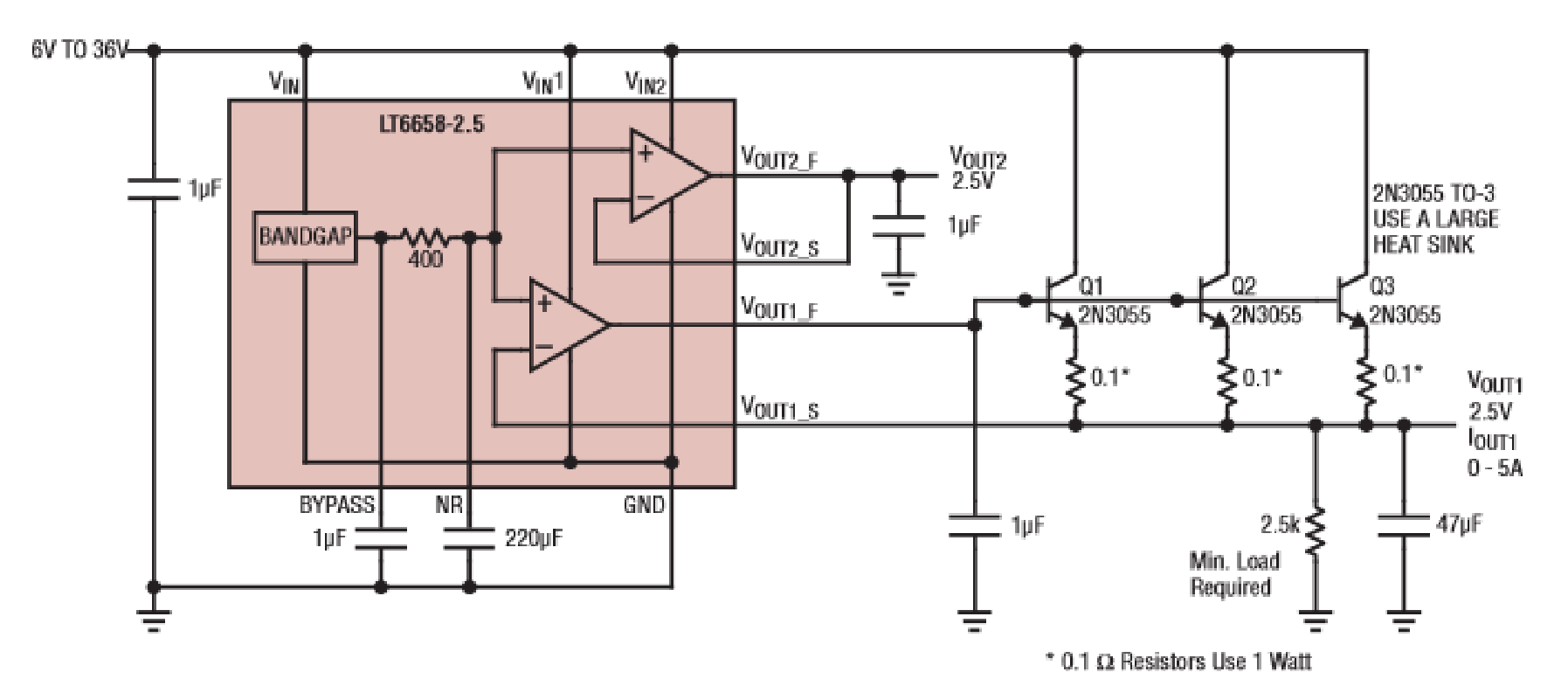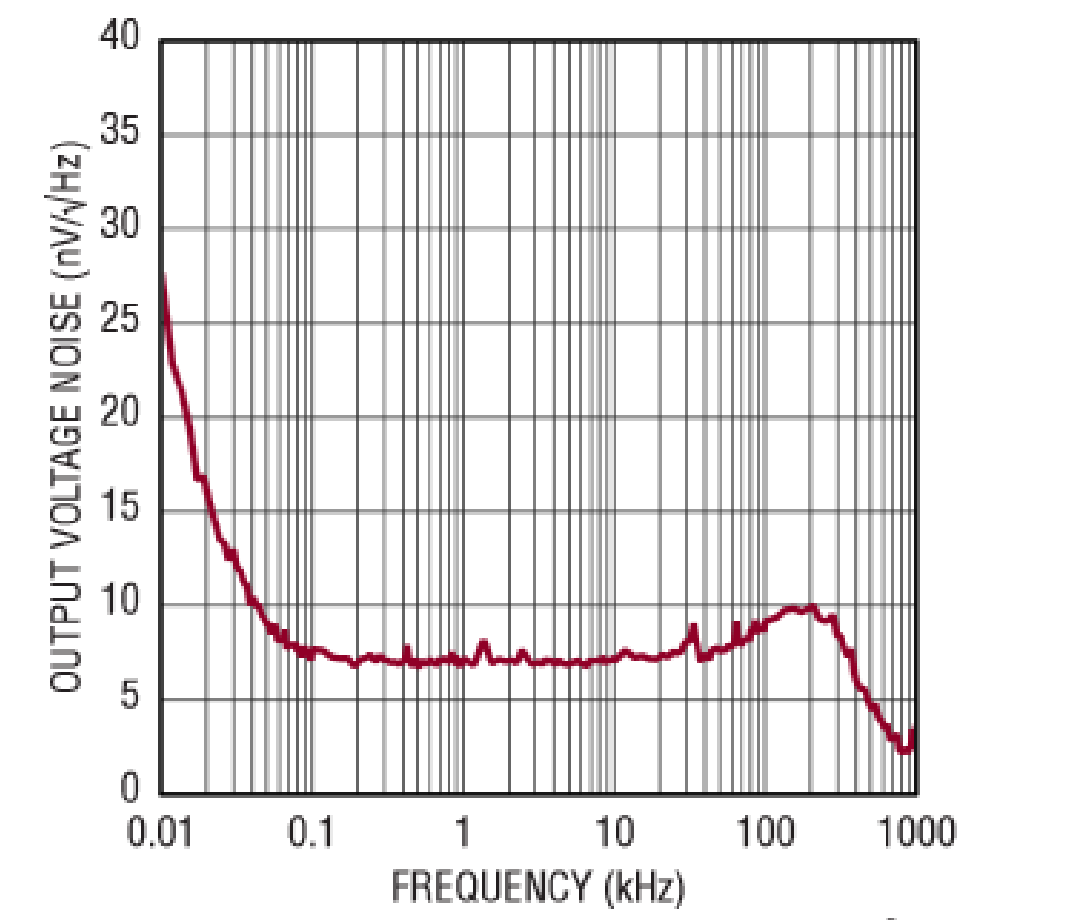# Build a Precision Low Noise 5A Power Supply

The LT6658-2.5V voltage reference has the specifications of a precision reference with dual outputs capable of 150mA and 50mA. This unprecedented combination of precision and power allows the unique ability to create a very precise high-current power supply with load regulation of less than 12ppm/A. Only a few additional components are required to accompany the LT6658.

A typical configuration of the LT6658-2.5V is shown below in Figure 1. The bandgap block endows the part with world class reference specifications. The 400Ω resistor sets up a low-pass filter that is completed by placing an arbitrarily large capacitor on the noise reduction (NR) pin. Finally the two outputs, VOUT1 and VOUT2, are buffered by low drift amplifiers with Kelvin sense inputs.

A 5A capability is achieved by adding three 2N3055 NPN transistors in parallel, as shown below in Figure 2. For 5A total output drive, each 2N3055 will pass 1.667A of current. Ballast resistors of value 0.1Ω balance power in the 2N3055s. Note that while the ballast resistors are only 0.1Ω, with 1.67A flowing through them they will dissipate 0.28W of power each. The LT6658 requires 2.5V of headroom to its Force outputs, and this circuit will require additional headroom of one VBE (approximately 0.7V) for the external NPNs along with the voltage drop across the ballast resistors. Calculating total power dissipated in the 2N3055s, 5A × (2.5V + 0.7V) = 16W. Thus, an adequate heat sink is required for the 2N3055 TO-3 packages.

To improve power efficiency, use an independent power supply for the output stage as shown in Figure 2. The output power supply can be lower to accommodate only the headroom required by the 2N3055s.Figure 2. Fixed 2.5V 5A Supply

This fixed-voltage supply is stable with a 1μF to 50μF capacitor on the VOUT1_F pin. Additional capacitance on the supply output at VOUT1_S can vary from a small capacitor to a large capacitor in the milli-farad range. This circuit cannot sink current from VOUT1_S. Sinking current is confined to the VOUT1_F pin. A 2.5kΩ external resistor keeps the loop active with a 1mA load. This resistor is not required if the load will always draw enough current to keep the circuit sourcing current.

Load regulation for the circuit above is shown below in Figure 3. At 5A the total error is less than 60ppm, resulting in a load regulation of less than 12ppm/A.

With a few small modifications, this circuit can be transformed into a variable power supply as shown in Figure 4. The LT6658 has a fixed output of 2.5V. To reduce the output range to 1V, a 267Ω resistor is placed on the NR pin to create a voltage-divider. A gain circuit around the output buffer is incorporated by adding a fixed 1kΩ and variable 4kΩ resistor. Note that the minimum voltage on the LT6658 supply pins is 4.25V on the VIN pin. The minimum voltage on VIN1 is 4.5V or VOUT1 + 2.5V + VBE whichever is higher.Figure 4. Precision Low Noise Variable 1V to 5V 5A Supply

To demonstrate the low noise capability, a 220μF capacitor is placed on the NR pin, as show below in Figure 5a. This rolls off the bandgap noise and reduces the noise density to about 7nV/√Hz. The output voltage noise plot of the 5-Ampere circuit is shown in Figure 5b.Figure 5a. Circuit used to demonstrate 5A noise performanceFigure 5b. Output Voltage Noise of the 5A Supply

The 2N3055 transistors exhibit a β of about 100, requiring 50mA of base drive well within the output drive specification of VOUT1.

Since the LT6658 has two outputs, the second output can maintain superior reference performance while the first output is configured as a high current power supply.

If two high current outputs are required, it is possible to configure the second output as a low noise high current power supply. Each buffer has a dedicated power supply pin resulting in excellent power supply rejection and very low channel-to-channel crosstalk.